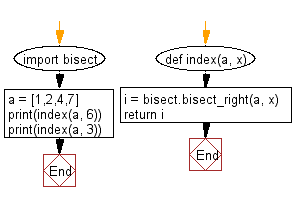﻿ Python Bisect: Locate the right insertion point for a specified value in sorted order - w3resource# Python Bisect: Locate the right insertion point for a specified value in sorted order

## Python Bisect: Exercise-2 with Solution

Write a Python program to locate the right insertion point for a specified value in sorted order.

Sample Solution:

Python Code:

``````import bisect
def index(a, x):
i = bisect.bisect_right(a, x)
return i

a = [1,2,4,7]
print(index(a, 6))
print(index(a, 3))
```
```

Sample Output:

```3
2
```

Flowchart:## Visualize Python code execution:

The following tool visualize what the computer is doing step-by-step as it executes the said program:

Python Code Editor:

What is the difficulty level of this exercise?

Test your Programming skills with w3resource's quiz.

﻿

## Python: Tips of the Day

How to make a chain of function decorators?

```from functools import wraps

def makebold(fn):
@wraps(fn)
def wrapped(*args, **kwargs):
return "<b>" + fn(*args, **kwargs) + "</b>"
return wrapped

def makeitalic(fn):
@wraps(fn)
def wrapped(*args, **kwargs):
return "<i>" + fn(*args, **kwargs) + "</i>"
return wrapped

@makebold
@makeitalic
def hello():
return "hello world"

@makebold
@makeitalic
def log(s):
return s

print hello()        # returns "<b><i>hello world</i></b>"
print hello.__name__ # with functools.wraps() this returns "hello"
print log('hello')   # returns "<b><i>hello</i></b>"
```

Ref: https://bit.ly/3cVz5iw